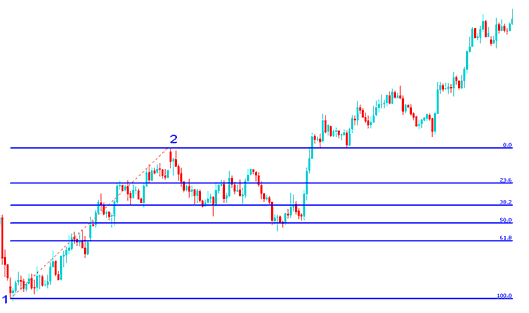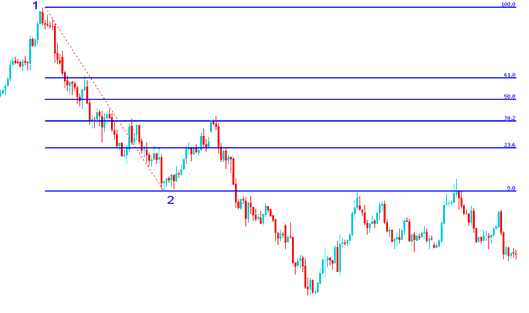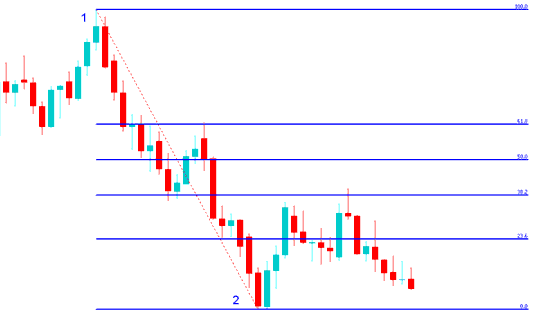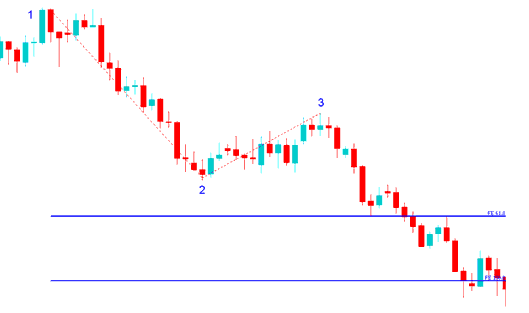Learn Gold Trading for Beginners Tutorials

# Fibonacci XAUUSD Exercises

These exercises will give more examples on retracement and expansion. The first exercise is to figure out the direction of the XAUUSD trend and then determine if the Fibonacci tool used is retracement or expansion and then figure how it is drawn then practice drawing them on a xauusd chart.

1. Figure out the direction of the XAUUSD trend
2. Determine if the Fibonacci is retracement or expansion
3. Figure how it is drawn
4. Practice drawing retracement or expansion on a xauusd chart.

After you have downloaded MetaTrader 4, Go to the menu "insert" at the top left corner, on the drop down menu, click Fibonacci and select either the option of retracement or expansion. Then draw it as explained in the previous lessons of Expansion and retracement. Then do the following exercises:

## Exercises 1:Exercises 2:Exercises 3:Exercises 4:Exercises 5:Hint: point 1 to point 2 follows the xauusd trend direction

Hint: Retracement has points 1 and 2

Hint: Expansion has point 1, 2 and 3